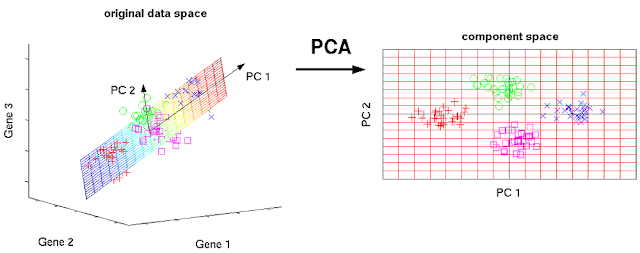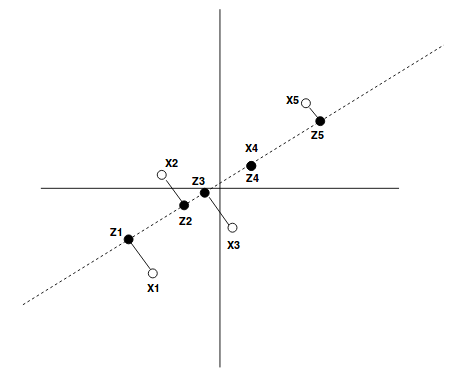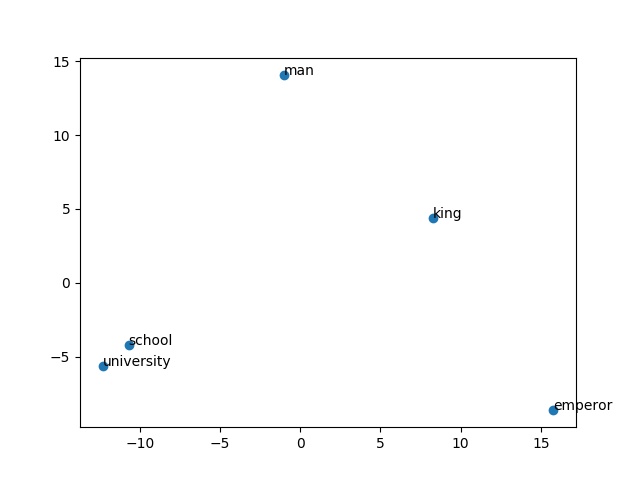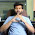## January 13, 2019

### A Layman’s Introduction to Principal Components[Source]

In this post, we will discuss about PCA - Principal Components Analysis, a classic algorithm that is being practiced since very long and continues to deliver desirable results.

What are dimensions?
In machine learning, dimensionality simply refers to the number of features (i.e. input variables) in your dataset.

Let’s say you want to predict the price of the house. Then what all parameters/features will you consider?
1. Area sq.ft
2. Locality
3. # Bedrooms
4. Internet speed
5. Distance from hospital
6. Distance from main market
7. etc .. etc
Just now itself we are dealing with 6–7 dimensional data for just predicting the house price. So, these things that matter while making any decisions are called dimensions.

What is high dimensional data?
For the purpose of visualization high dimension can be any number of dimension above 3 to 4. Whereas, in general, I personally have found reduction working really well when visualizing the word embeddings which are usually in the order of few 100s.

Why reduce the dimensions?
• Large dimensions are difficult to train on, need more computational power and time.
• Visualization is not possible with very large dimensional data.
• Loading very high dimensional data can be an issue with limited storage space in-memory.
• It can be used to reduce the dimension of the features, potentially leading to better performance for the learning algorithm by removing redundant, obsolete and highly correlated features.

Always Remember
As a thumb rule, you should always do feature standardization before applying PCA to any dataset. Standardizing the features so that they are centered around 0 with a standard deviation of 1 is not only important if we are comparing measurements that have different units, but it is also a general requirement for many machine learning algorithms. All this because, we would want all the scale of measurements to be treated on the same scale.

Under the hood
PCA is a variance maximizer. It projects the original data onto the directions where variance is maximum.

Variance is the measure of how spread out the data is.2D to 1D data transformation

X(i) where i in [1,2,3,4,5] are the original data points in a 2-D space. Then Z(i) where i in [1,2,3,4,5] are the projected points on a 1-D space (Line). We chose the line going from -xy to +xy (dotted one) because the data is most spread in this direction. Now, for all the points in 2-D space X(i) we map them to 1-D space/component Z(i).

Let’s do one
We will be using scikit-learn for this experiment. People who are wondering what scikit-learn it read this. It is very easy to apply this statistical technique in python.Thanks to the community
Here, we will be dealing with a curated dataset just for the purpose of this snippet.

`````` from sklearn.decomposition import PCA
import matplotlib.pyplot as plt
from sklearn.preprocessing import scale
data = open('custom_embed.csv', 'rb')
labels, dimensions = [], []
for line in data:
line = line.split(",")
lab = line.strip()
dim = [float(j.strip()) for j in line.split()]
labels.append(lab)
dimensions.append(dim)
# scaling the values
X = scale(dimensions)
pca = PCA(n_components=2)
pca.fit(X)
X1=pca.fit_transform(X)
X1 = X1.tolist()
# plotting
x = [i for i in X1]
y = [i for i in X1]
n = ['king', 'school', 'university', 'man', 'emperor']
fig, ax = plt.subplots()
ax.scatter(x, y)
for i, txt in enumerate(n):
ax.annotate(txt, (x[i],y[i]))
plt.show()  ``````Embedding Visualization in 2-D

It seems that we are successful in preserving the semantic properties of words as and when they are used even after reducing the dimension space from 300 to 2.
I have tried to keep this blog simple and intuitive as much possible. For in-depth details of the algorithms see this

Feel free to share comment your thoughts on the same. — Thanks

#### 2 comments:

1.Nice read!

1.Please share your valuable feedback. It will help and me and community grow.